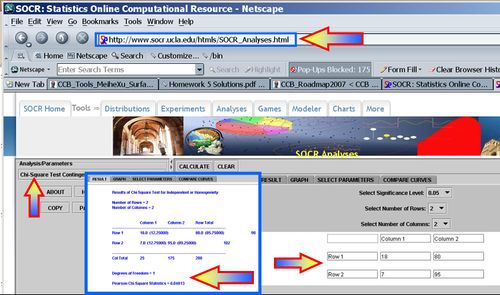# Difference between revisions of "AP Statistics Curriculum 2007 Contingency Indep"

## General Advance-Placement (AP) Statistics Curriculum - Contingency Tables: Independence and Homogeneity

### Contingency Tables: Independence and Homogeneity

The chi-square test may also be used to assess independence and association between variables.

## Motivational example

Suppose 200 randomly selected cancer patients were asked if their primary diagnosis was Brain cancer and if they owned a cell phone before their diagnosis. The results are presented in the table below.

Suppose we want to analyze the association, if any, between brain cancer and cell phone use. The 2x2 table below lists two possible outcomes for each variable (each variable is dichotomous). We have the following population parameters:

P(CP|BC) = true probability of owning a cell phone (CP) given that the patient had brain cancer (BC). This chance may be estimated by P(CP|BC) = 0.72.
P(CP|NBC) = true probability of owning a cell phone given that the patient had another cancer, which is estimated by P(CP|NBC) = 0.46
 Brain cancer Yes No Total Cell Phone Use Yes 18 80 98 No 7 95 102 Total 25 175 200

Does it seem like there is an association between brain cancer and cell phone use? Of the brain cancer patients 18/25 = 0.72, owned a cell phone before their diagnosis. P(CP|BC) = 0.72, estimated probability of owning a cell phone given that the patient has brain cancer.

Of the other cancer patients, 80/175 = 0.46, owned a cell phone before their diagnosis. P(CP|NBC) = 0.46, estimated probability of owning a cell phone given that the patient has another cancer.

## Calculations

• The hypotheses:

$H_o$: there is no association between variable 1 and variable 2 (independence)

P(BC|CP)=P(BC), that is brain-cancer (BC) is independent of cell-phone (CP) usage.

$H_a$: there is an association between variable 1 and variable 2 (dependence)

$P(BC|CP)={P(BC \cap CP) \over P(CP) } \not= P(BC).$
• Test statistics:

The test statistic:

$\chi_o^2 = \sum_{all-categories}{(O-E)^2 \over E} \sim \chi_{(df)}^2$, where df = (# rows – 1)(# columns – 1).

Expected cell counts can be calculated by
$E = { (row\_total)(column\_total)\over grand-total}$
• Results:

For the Brain-cancer and cell-phone usage data we have: $\chi_o^2 = {(18-12.25)^2\over 12.25} + {(7-12.75)^2\over 12.75} + {(80-85.75)^2\over 85.75}+ {(95-89.25)^2\over89.25}$

$\chi_o^2 = =6.048 \sim \chi_{(df=1)}^2$
P-value$P(\chi_1^2 > \chi_o^2)= 0.014306.$ and we can reject the null hypothesis at $$\alpha=0.05$$.